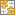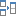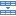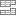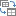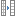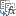# How to use LEN function in Excel to count characters in a cell

Are you looking for an Excel formula to count characters in a cell? If so, then you have certainly landed up on the right page. This short tutorial will teach you how you can use the LEN function to count characters in Excel, with or without spaces.

Of all Excel functions, LEN is arguably the easiest and most straightforward one. The name of the function is easy to remember, it's nothing else but the first 3 characters of the word "length". And it is what the LEN function actually does - returns the length of a text string, or length of a cell.

To put it differently, you use the LEN function in Excel to count all characters in a cell, including letters, numbers, special characters, and all spaces.

In this short tutorial, we are going to cast a quick look at the syntax first, and then have a closer look at some useful formula examples to count characters in your Excel worksheets.

## Excel LEN function

The LEN function in Excel counts all characters in a cell, and returns the string length. It has just one argument, which is obviously required:

=LEN(text)

Where text is the text string for which you want to count the number of characters. Nothing could be easier, right?

Below you will find a couple of simple formulas to get the basic idea of what the Excel LEN function does.

`=LEN(123)` - returns 3, because 3 numbers are supplied to the text argument.

`=LEN("good")` - returns 4, because the word good contains 4 letters. Like any other Excel formula, LEN requires enclosing text strings double quotes, which are not counted.

In your real-life LEN formulas, you are likely to supply cell references rather than numbers or text strings, to count characters in a specific cell or a range of cells.

For instance, to get the length of text in cell A1, you'd use this formula:

`=LEN(A1)`

More meaningful examples with detailed explanations and screenshots follow below.

## How to use LEN function in Excel - formula examples

At first sight, the LEN function looks so simple that hardly requires any further explanation. However, there are some useful tricks that could help you tweak your Excel Len formula for your specific needs.

### How to count all characters in a cell (including spaces)

As already mentioned, the Excel LEN function counts absolutely all characters in a specified cell, including all spaces - leading, trailing spaces, and spaces between words.

For example, to get the length of cell A2, you use this formula:

`=LEN(A2)`

As shown in the below screenshot, our LEN formula counted 36 characters including 29 letters, 1 number, and 6 spaces.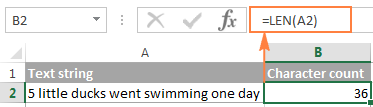#### Count characters in multiple cells

To count characters in multiple cells, select the cell with your Len formula and copy it to other cells, for example by dragging the fill handle. For the detailed instructions, please see How to copy formula in Excel.

As soon as the formula is copied, the LEN function will return the character count for each cell individually.

And again, let me draw your attention that the LEN function counts absolutely everything including letters, numbers, spaces, commas, quotes, apostrophes, and so on: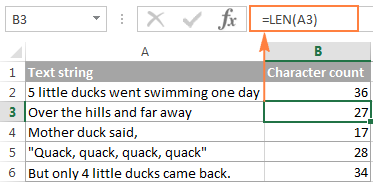Note. When copying a formula down the column, be sure to use a relative cell reference like `LEN(A1)`, or a mixed reference like `LEN(\$A1)` that fixes only the column, so that your Len formula will properly adjust for the new location. For more information, please check out Using absolute and relative cell references in Excel.

#### Count a total number of characters in several cells

The most obvious way to get the total number of characters in several cells is add up a few LEN functions, for example:

`=LEN(A2)+LEN(A3)+LEN(A4)`

Or, use the SUM function to total the character counts returned by LEN formulas:

`=SUM(LEN(A2),LEN(A3),LEN(A4))`

Either way, the formula counts the characters in each of the specified cells and returns the total string length: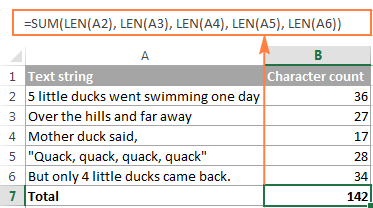This approach is undoubtedly easy-to-understand and easy-to-use, but it's not the best way to count characters in a range consisting of, say, 100 or 1000 cells. In this case, you'd better use the SUM and LEN functions in an array formula, and I will show you an example in our next article.

### How to count characters excluding leading and trailing spaces

When working with big worksheets, a common problem is leading or trailing spaces, i.e. extra spaces at the beginning or at the end of the items. You would hardly notice them on the sheet, but after you've faced them a couple of times, you learn to beware of them.

If you suspect there are a few invisible spaces in your cells, the Excel LEN function is a great help. As you remember, it includes all spaces in a character count: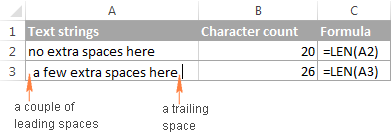To get the string length without leading and trailing spaces, simply embed the TRIM function in your Excel LEN formula:

`=LEN(TRIM(A2))`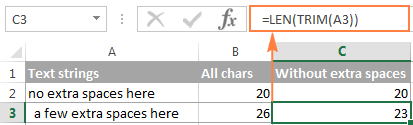### How to count the number of characters in a cell excluding all spaces

If your aim is to get the character count without any spaces whether leading, trailing or in-between, you would need a bit more complex formula:

`=LEN(SUBSTITUTE(A2," ",""))`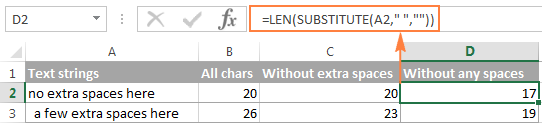As you probably know, the SUBSTITUTE function replaces one character with another. In the above formula, you replace a space (" ") with nothing, i.e. with an empty text string (""). And because you embed SUBSTITUTE in the LEN function, the substitution is not actually made in cells, it just instructs your LEN formula to calculate the length of string without any spaces.

You can find a more detailed explanation of the Excel SUBSTITUTE function here: Most popular Excel functions with formula examples.

### How to count number of characters before or after a given character

Occasionally, you may need to know the length of a certain part of a text string, rather than count the total number of characters in a cell.

Supposing, you have a list of SKUs like this: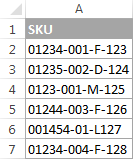And all valid SKUs have exactly 5 characters in the first group. How do you spot invalid items? Yep, by counting the number of characters before the first dash.

So, our Excel length formula goes as follows:

`=LEN(LEFT(\$A2, SEARCH("-", \$A2)-1))`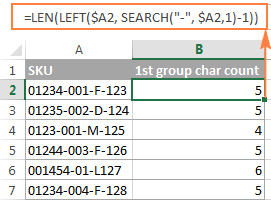And now, let's break down the formula so that you can understand its logic.

• You use the SEARCH function to return the position of the first dash ("-") in A2:
`SEARCH("-", \$A2)`
• Then, you use the LEFT function to return that many characters starting on the left side of a text string, and subtract 1 from the result because you don't want to include the dash:
`LEFT(\$A2, SEARCH("-", \$A2,1)-1))`
• And finally, you have the LEN function to return the length of that string.

As soon as the character count is there, you may want to take a step further, and highlight invalid SKUs by setting up a simple conditional formatting rule with a formula like `=\$B2<>5:`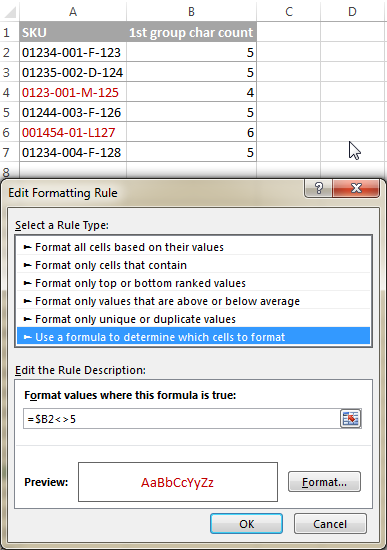Or, you can identify invalid SKUs by embedding the above LEN formula in the IF function:

`=IF(LEN(LEFT(\$A2, SEARCH("-", \$A2)-1))<>5, "Invalid", "")`

As demonstrated in the below screenshot, the formula perfectly identifies invalid SKUs based on a string length, and you don't even need a separate character count column: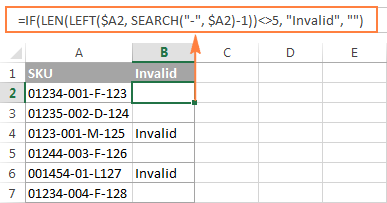In a similar manner, you can use the Excel LEN function to count the number of characters after a specific character.

For example, in a list of names, you may want to know how many characters the Last Name contains. The following LEN formula does the trick:

`=LEN(RIGHT(A2,LEN(A2)-SEARCH(" ",A2)))`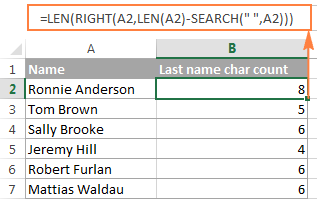How the formula works:

• First, you determine the position of a space (" ") in a text string by using the SEARCH function:
`SEARCH(" ",A2)))`
• Then, you calculate how many characters follow the space. For this, you subtract the space position from the total string length:
`LEN(A2)-SEARCH(" ",A2)))`
• After that, you have the RIGHT function to return all characters after the space.
• And finally, you use the LEN function to get the length of string returned by the RIGHT function.

Please note, for the formula to work correctly, each cell should contain just one space, i.e. only the First and Last name, with no middle names, titles or suffixes.

Well, this is how you use LEN formulas in Excel. If you want to have a closer look at the examples discussed in this tutorial, you are welcome to download a sample Excel LEN workbook.

In the next article, we are going to explorer other capabilities of the Excel LEN function, and you will learn a few more useful formulas to count characters in Excel:

In the meantime, I thank you for reading and hope to see you on our blog soon!

### 19 responses to "How to use LEN function in Excel to count characters in a cell"

1.Amit says:

Very nice article Svetlana.. keep it up !

2.DIke says:

Please how do you count the no.of alphabets alone, supposing the characters contain alphabets

3.Francis says:

Hi

I have a string

John Thomas 215
Need to get... John Thomas only with no spaces etc.
Formulas do not recognise the last space as the one before the numbers 215, do not know why.
So using the formulas regarding "last space" does not work for this.

I also use ; and not , in my formulas.

Desperate Francis

•Steve says:

Assuming the text John Smith 215 is in cell D3:
=SUBSTITUTE(D3," ","")
This function will eliminate the blank spaces that you mentioned.

•Steve says:

And if you were to stack the item as Kenneth indicated then:
=LEN(SUBSTITUTE(D3," ",""))

4.Kenneth says:

Thank you very, very, very much.
This information is invaluable, priceless, totally useful and completely simple.

I have been SEARCHING for a line of EXCEL code that would count the characters in a cell minus the spaces.

For example suppose cell A3 contained "1 2 3 4".
=LEN(A3) would return 7 as the answer.
=LEN(SUBSTITUTE(A3," ","")) would return 4 as the answer. That is what I was looking for.

I found other sites that suggested VBA language/code to do the same thing.

=LEN(SUBSTITUTE(A3," ",""))

Just that piece of information makes your site great. I will visit you again.

Thank you.
Kenneth from Virginia

5.San Bunna Svay says:

Len function count wrong number in the cell such as in each cell has only 15 digits but I used LEN function to count them, it has shown 17 digits.
Thanks so much for your solving.
San Bunna Svay

6.Nelson says:

Hey

I have a set of number in three columns
1 2 3 4
1 3 5 7 9
4 5 7

I want each number to be in is respective cell in a column after converting

7.SHARAVAN V says:

i need know how many emp id in cell

for exp: 70000001,70000002,70000004,70000003,70000005

•Doug says:

Sharavan:
Where the IDs are in cell R18, the formula is:
=LEN(TRIM(R18))-LEN(SUBSTITUTE(TRIM(R18),",",""))+1

8.Harinder Singh says:

What an explanation. This is first site i have seen that someone is explaining the things so easily and all information regarding the particular thing is available. I like your article and site. Thank you very much. Doing nice work

Stay Blessed

9.Sergej P. says:

Hi Svetlana,

I have different types of data in a cell that I'd like to get an accurate count of. For example, C8 contains this: C111,C125,C132,C139,C146,C203,C219,C245,C251,C271,C293-C297

Is it possible to get an accurate count of values separated by both a comma and a dash? C8 contains 11 values separated by a comma (C293-C297 is counted as 1), but I'm curious if the last value (C293-C297) can be counted as C293, C294, C295, C296, C297, giving me the total of 15 that I'm looking for?

Thank you very much for all your great work!
-Sergej

10.Ivor Shaer says:

Hi Svetlana,
How would you write a macro to replace "=LEN(LEFT(\$A2, SEARCH("-", \$A2)-1))"
I tried: Range("D3").FormulaR1C1 = Len(Left(Selection.Offset(-1, 0), Find(".", Selection.Offset(-1, 0)))) to get the number of characters in Range("C3").
Does not work!
Thanks
Ivoir

11.SANTANU PRADHAN says:

in an excel sheet there is a number 9556127584 but when we put len formula then it shows 11nos why

12.Hammad says:

If We have number like 000.0253.3265.235 length may be vary. We need to get number with except to the first value up to the decimal, and the last value from the decimal onward.
Result Should Be: 0253.3265

•Alexander Trifuntov (Ablebits.com Team) says:

If I understand your task correctly, the following formula should work for you:

=LEFT(SUBSTITUTE(B2,"000.",""), LEN(SUBSTITUTE(B2,"000.",""))-4)

I hope this will help

13.Henry says:

Hi,

How would you count the length of characters in a cell that contain 'ellipsis' dots in excel? e.g.
M...... ; ideally this should count as 7 character but excel counts 3 continuous dots as 1.

Regards
Henry

•Alexander Trifuntov (Ablebits.com Team) says:

Hello!
Check out Auto Correct Options in Excel. Whenever you enter three dots, they are automatically replaced with the special Ellipsis character. Therefore, the number of characters in the text is reduced.

Ultimate Suite for Microsoft Excel